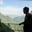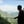Trusted answers to developer questions
Trusted Answers to Developer Questions

Related Tags

pandas
dataframe
communitycreator

# What is the pandas DataFrame.sub() method?AKASH BAJWA

Grokking Modern System Design Interview for Engineers & Managers

Ace your System Design Interview and take your career to the next level. Learn to handle the design of applications like Netflix, Quora, Facebook, Uber, and many more in a 45-min interview. Learn the RESHADED framework for architecting web-scale applications by determining requirements, constraints, and assumptions before diving into a step-by-step design process.

## Overview

The pandas software library is written for Python and is mostly used for data analysis. It works as a data manipulation module.

The pandas DataFrame is a two-dimensional tabular data structure in which data is aligned in a tabular form in rows and columns.

The pandas Dataframe consists of three principal components:

• Principal Data
• Rows (placed left to right horizontally)
• Columns (placed top to bottom vertically)

## The pandas DataFrame.sub() method

Here, sub() means subtraction, and this method performs subtraction operations on data frames. It is an element-wise operation and works like a binary subtraction ( - ) operator.

### Syntax

DataFrame.sub (other, axis = 'columns', level = None, fill_value = None)

### Parameters

It has the following argument values:

• other: This parameter is a single or multiple element data structure or list-like object. It can be a DataFrame, series, sequence, scalar, or a constant.
• axis: This is used for deciding the axis on which the operation is applied. Whether to compare by the index (0 or ‘index’) or columns (1 or ‘columns’) that is {0 or ‘index’, 1 or ‘columns’}.
• level: This parameter is used to broadcast across a level and matching index values on the passed MultiIndex level. It is either a number or a label that indicates where to compare.
• fill_value: This parameter is a number or None. It specifies what to do with NaN values before subtracting. If data in both corresponding DataFrame locations is missing, the result will be missing.

Here, the first parameter is required and the other three are optional.

### Return value

This method returns a dataFrame in the result obtained by subtraction of two DataFrames, or a DataFrame division with a scaler.

### Explanation

The first thing for implementation is to import pandas. Here we are importing pandas as pd. So, pd will be used in place of panda in the entire program.

### Subtraction of a single value from all entries of a DataFrame

# importing pandas as pdimport pandas as pd# Creating a dataframe with four observationsdf= pd.DataFrame({"ClassA":[100,50,10],				    "ClassB":[50,20,30],				    "ClassC":[70,70,25],				    "ClassD":[150,300,0]})# Print the dataframeprint(df)print()#subtractin of 10 from each and every valueprint(df.sub(10))
• Lines 4–7: We create a DataFrame including dictionaries having classes as keys.
• Line 9: We print the DataFrame.
• Line 12: Here the multiplication method is used that is df.sub(10)

When a single parameter (10) is passed, it will be subtracted from every entry of the DataFrame.

### Subtraction of distinct values from different data sets in DataFrame

# Subtract these elements from the respective classprint(df.sub([20, 10, 5, 1], axis='columns'))

We are subtracting 20 from the first class, 10 from the second class, 5 from the third class, and 1 from the fourth class where the axis is columns.

### Series subtraction w.r.t index in DataFrame

# importing pandas as pdimport pandas as pd# Creating a dataframe with three observationsdf= pd.DataFrame({"ClassA":[100,50,10],				    "ClassB":[50,20,30],				    "ClassC":[70,70,25],})# Print the dataframeprint(df)print()# subtracting with series type dataprint(df.sub(pd.Series([5, 10, 2], index=[0,1,2]), axis='index'))

### Explanation

Here, we have three elements in series 5, 10, and 20 and indexes as 0, 1, and 2. Since the method is applied index wise axis = index, the result is obtained in a way that the first series element 5 will be subtracted from each value of the first index which is 0. The next series element 10, will be subtracted from each value of index 1 and so on.

We can perform a variety of subtractions on one or more DataFrames just by changing parameters in different ways by using the DataFrame.sub() method. In the case of any NaNmissing value value, we can use the fill_value parameter and assign it by the value we want written in place of the empty or missing values in the data instead of NaN.

RELATED TAGS

pandas
dataframe
communitycreator

CONTRIBUTORAKASH BAJWA

Grokking Modern System Design Interview for Engineers & Managers

Ace your System Design Interview and take your career to the next level. Learn to handle the design of applications like Netflix, Quora, Facebook, Uber, and many more in a 45-min interview. Learn the RESHADED framework for architecting web-scale applications by determining requirements, constraints, and assumptions before diving into a step-by-step design process.

Keep Exploring

Learn in-demand tech skills in half the time